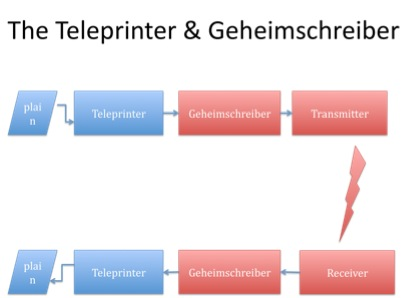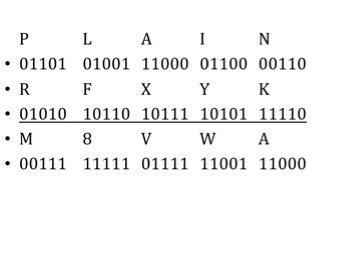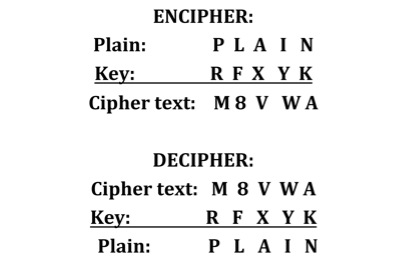The Lorenz Cipher and the teleprinter

The first illustration shows schematically how the Geheimschreiber (‘secret writer’) cipher device is attached to a teleprinter and automatically enciphers the message output from the teleprinter (and automatically deciphers the incoming message passing it on to a teleprinter for printing).The teleprinter used the ‘Baudot code’ which allocates one of 32 combinations of five on/off units to letters of the alphabet and numbers (eg N is 00110, R is 01010, I is 01100 and so on). A ‘shift’ function increased the number of characters that could be encoded to 60.

The ‘Vernam cipher’ was used by the Geheimschreiber to encipher the message encoded in Baudot code format.

There are four basic rules: 0+0=0, 0+1=1, 1+0=1 & 1+1=0.

0 0 1 1
0 1 0 1 +
0 1 1 0

Example: combine the Baduot code for ’N’ with that for ‘R’ producing ‘I’:

N - 0 0 1 1 0
R -
0 1 0 1 0 +
I - 0 1 1 0 0

The extended example below combines the random key
‘ R F X Y K’ with the word ‘P L A I N’,
giving the enciphered character stream M 8 V W AThe way the Vernam cipher works means that exactly the same operation is used for deciphering the message. The same key is combined with the enciphered text as shown below (showing only the alphabetic character rather than the five bits).